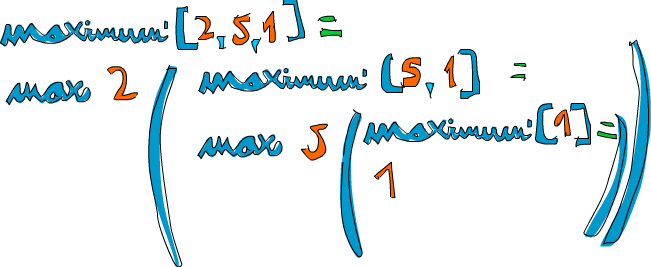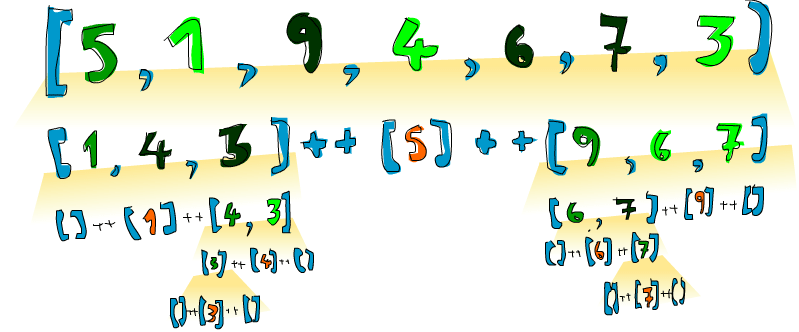# 递归

## 你好，递归！## 实作 Maximum

`maximum` 函数取一组可排序的 List（属于 Ord Typeclass） 做参数，并回传其中的最大值。想想，在命令式风格中这一函数该怎么实现。很可能你会设一个变量来存储当前的最大值，然后用循环遍历该 List，若存在比这个值更大的元素，则修改变量为这一元素的值。到最后，变量的值就是运算结果。唔！描述如此简单的算法还颇费了点口舌呢！

``````maximum' :: (Ord a) => [a] -> a
maximum' [] = error "maximum of empty list"
maximum' [x] = x
maximum' (x:xs)
| x > maxTail = x
| otherwise = maxTail
where maxTail = maximum' xs
``````

``````maximum' :: (Ord a) => [a] -> a
maximum' [] = error "maximum of empty list"
maximum' [x] = x
maximum' (x:xs) = max x (maximum' xs)
``````## 来看几个递归函数

``````replicate' :: (Num i, Ord i) => i -> a -> [a]
replicate' n x
| n <= 0    = []
| otherwise = x:replicate' (n-1) x
``````

``````*Note*: Num 不是 Ord 的子集, 表示数字不一定得拘泥于排序, 这就是在做加减法比较时要将 Num 与 Ord 型别约束区别开来的原因.
``````

``````take' :: (Num i, Ord i) => i -> [a] -> [a]
take' n _
| n <= 0   = []
take' _ []     = []
take' n (x:xs) = x : take' (n-1) xs
```````reverse` 函数简单地反转一个 List, 动脑筋想一下它的边界条件! 该怎样呢? 想想...是空 List! 空 List 的反转结果还是它自己. Okay, 接下来该怎么办? 好的, 你猜的出来. 若将一个 List 分割为头部与尾部, 那它反转的结果就是反转后的尾部与头部相连所得的 List.

``````reverse' :: [a] -> [a]
reverse' [] = []
reverse' (x:xs) = reverse' xs ++ [x]
``````

Haskell 支持无限 List，所以我们的递归就不必添加边界条件。这样一来，它可以对某值计算个没完, 也可以产生一个无限的数据结构，如无限 List。而无限 List 的好处就在于我们可以在任意位置将它断开.

`repeat` 函数取一个元素作参数, 回传一个仅包含该元素的无限 List. 它的递归实现简单的很, 看:

``````repeat' :: a -> [a]
repeat' x = x:repeat' x
``````

`zip` 取两个 List 作参数并将其捆在一起。`zip [1,2,3] [2,3]` 回传 `[(1,2),(2,3)]`, 它会把较长的 List 从中间断开, 以匹配较短的 List. 用 `zip` 处理一个 List 与空 List 又会怎样? 嗯, 会得一个空 List, 这便是我们的限制条件, 由于 `zip` 取两个参数, 所以要有两个边缘条件

``````zip' :: [a] -> [b] -> [(a,b)]
zip' _ [] = []
zip' [] _ = []
zip' (x:xs) (y:ys) = (x,y):zip' xs ys
``````

``````elem' :: (Eq a) => a -> [a] -> Bool
elem' a [] = False
elem' a (x:xs)
| a == x    = True
| otherwise = a `elem'` xs
``````

## "快速"排序``````quicksort :: (Ord a) => [a] -> [a]
quicksort [] = []
quicksort (x:xs) =
let smallerSorted = quicksort [a | a <- xs, a <= x]
biggerSorted = quicksort [a | a <- xs, a > x]
in smallerSorted ++ [x] ++ biggerSorted
``````

``````ghci> quicksort [10,2,5,3,1,6,7,4,2,3,4,8,9]
[1,2,2,3,3,4,4,5,6,7,8,9,10]
ghci> quicksort "the quick brown fox jumps over the lazy dog"
" abcdeeefghhijklmnoooopqrrsttuuvwxyz"
``````

booyah! 如我所说的一样! 若给 `[5,1,9,4,6,7,3]` 排序，这个算法就会取出它的头部，即 5。 将其置于分别比它大和比它小的两个 List 中间，得 `[1,4,3] ++  ++ [9,6,7]`, 我们便知道了当排序结束之时，5会在第四位，因为有3个数比它小每，也有三个数比它大。好的，接着排 `[1,4,3]``[9,6,7]`, 结果就出来了！对它们的排序也是使用同样的函数，将它们分成许多小块，最终到达临界条件，即空 List 经排序依然为空，有个插图：## 用递归来思考